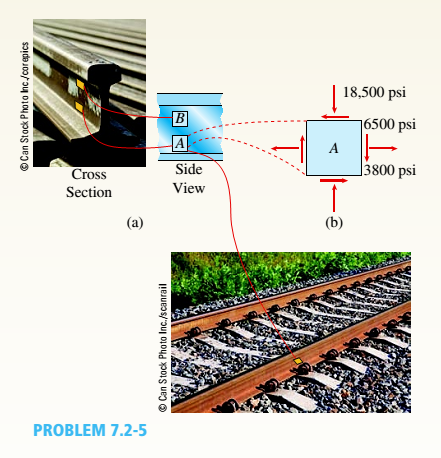# The stresses acting on element A on the web of a train rail (sec figure part a) a re found to be 6500 psi tension in the horizontal direction and 18,500 psi compression in the vertical direction (see figure part b), Also, shear stresses with a magnitude of 3800 psi act in the directions shown. Determine the stresses acting on an clement oriented at a counterclockwise angle of 30° from the horizontal Show these stresses on a sketch of an element oriented at this angle### Mechanics of Materials (MindTap Co...

9th Edition
Barry J. Goodno + 1 other
Publisher: Cengage Learning
ISBN: 9781337093347
Chapter 7, Problem 7.2.5P
Textbook Problem
1 views

## The stresses acting on element A on the web of a train rail (sec figure part a) a re found to be 6500 psi tension in the horizontal direction and 18,500 psi compression in the vertical direction (see figure part b), Also, shear stresses with a magnitude of 3800 psi act in the directions shown.Determine the stresses acting on an clement oriented at a counterclockwise angle of 30° from the horizontal Show these stresses on a sketch of an element oriented at this angleTo determine

Stress acting on an element.

Show these stress on a sketch of an element oriented at this angle.

### Explanation of Solution

Write the expression for normal stress acting along the x direction

σx=(σx+σy2)+(σxσy2)cos2θ+τxysin2θ        …… (I)

Here, stress acting along the x direction is σx, stress is acting along y direction σy,shear stress acting along xy is τxy, angle oriented on an element is θ.

Write the expression for normal stress acting on the y1axis inclined to the original axis.

σy=(σx+σy2)(σxσy2)cos2θτxysin2θ        …… (II)

Write the expression for shear stress acting on the x1y1plane inclined to the original axis.

τxy=(σxσy2)sin2θ+τxycos2θ        …… (III)

Calculation:

The following figure shows the stress acting on an element at an angle θ=30°.

Figure-(1)

The following figure shows that stress acting on an element.

Figure-(2)

Substitute, 6500psifor σx, 18500psifor σy, and 3800psifor τxy, 30°for θin the Equation (I)

σx=(6500psi18500psi2)+(6500psi+18500psi2)cos(2×30°)(3800psi)sin(2×30°)=(12000psi2)+(25000psi2)×(0.5)(3800psi)(0.866025404)=6000psi+12500psi×(0

### Still sussing out bartleby?

Check out a sample textbook solution.

See a sample solution

#### The Solution to Your Study Problems

Bartleby provides explanations to thousands of textbook problems written by our experts, many with advanced degrees!

Get Started

Find more solutions based on key concepts
When would a radial-arm drill press most likely be used?

Precision Machining Technology (MindTap Course List)

What does it mean if a bond is callable

Cornerstones of Financial Accounting

What is network footprinting?

Principles of Information Security (MindTap Course List)

What is a relationship, and what three types of relationships exist?

Database Systems: Design, Implementation, & Management

What is structural independence, and why is it important?

Database Systems: Design, Implementation, & Management

Calculate the attractive force between two students with masses of 70 kg and 80 kg. The students are standing a...

Engineering Fundamentals: An Introduction to Engineering (MindTap Course List)

Define an actor, and provide three examples.

Systems Analysis and Design (Shelly Cashman Series) (MindTap Course List)

Explain why a retailer would use RFID technology, and list uses for magstripe readers.

Enhanced Discovering Computers 2017 (Shelly Cashman Series) (MindTap Course List)

Why is the torch tip raised as the cutting lever is depressed when cutting a hole?

Welding: Principles and Applications (MindTap Course List)

If your motherboard supports ECC DDR3 memory, can you substitute non-ECC DDR3 memory?

A+ Guide to Hardware (Standalone Book) (MindTap Course List)